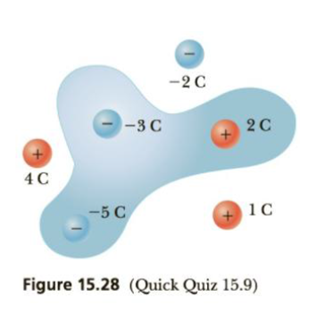Chapter 15.8, Problem 15.9QQ

Chapter
Section
Textbook Problem

Find the electric flux through the surface in Figure 15.28. Assume all charges in the shaded area are inside the surface. (a) −(3 C)/ϵ0 (b) (3 C)/ϵ0 (c) 0 (d) −(6C)/ϵ0To determine
The electric flux through the surface.

Explanation

From Gauss’s law, the magnitude of flux is given by,

ϕE=qnetε0 (I)

• qnet is the net charge enclosed by the Gaussian surface.
• ε0 is the permittivity of free space.

Conclusion:

The net charge enclosed by the Gaussian surface is,

qnet=(3C)+(5C)+(2

Still sussing out bartleby?

Check out a sample textbook solution.

See a sample solution

The Solution to Your Study Problems

Bartleby provides explanations to thousands of textbook problems written by our experts, many with advanced degrees!

Get Started